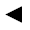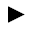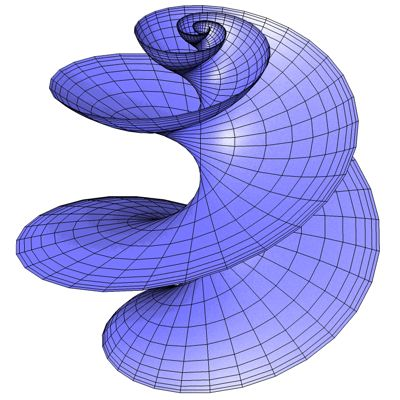﻿ math handbook calculator - Fractional Calculus Computer Algebra System software+++=[back]

## 3.22. Hyperbolic Helicoid

[next]The Hyperbolic Helicoid [ 6 ] is represented by the following equations.

 x = sinh(v) cos(a u)/(1+cosh(u) cosh(v)) 3-80 y = sinh(v) sin(a u)/(1+cosh(u) cosh(v)) 3-81 z = cosh(v) sinh(u)/(1+cosh(u) cosh(v)) 3-82

The constant a determines the appearance of the figure.

To represent the area, the two parameters u and v can assume the following values ​​(domain of definition), for example.

 u is an element from the set of numbers [-4, 4] v is an element from the number set [-4, 4]

The Hyperbolic Helicoid is an open figure, so the domain of definition can be changed in the plugin.Fig. 26